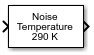Apply receiver thermal noise to complex signal

• Library:
• Communications Toolbox / RF Impairments

•## Description

The Receiver Thermal Noise block applies receiver thermal noise to a complex signal. The block simulates the effects of thermal noise on a complex signal. The Specification method parameter enables specification of the thermal noise based on the noise temperature, noise figure, or noise factor.

## Ports

### Input

expand all

Complex signal, specified as a scalar or column vector.

Data Types: `double` | `single`
Complex Number Support: Yes

### Output

expand all

Output signal, returned as a scalar or column vector. This output is the same dimension and data type as the input signal.

## Parameters

expand all

Thermal noise specification method, specified as one of these options.

• `Noise temperature` specifies the noise in kelvins.

• `Noise figure` specifies the added receiver noise in dB relative to an input noise temperature of 290 K. The noise figure is the decibel equivalent of the noise factor.

• `Noise factor` specifies the added receiver noise relative to an input noise temperature of 290 K. The noise factor is the linear equivalent of the noise figure.

Noise temperature in kelvins, specified as a scalar.

Tunable: Yes

#### Dependencies

To enable this parameter, set the Specification method parameter to ```Noise temperature```.

Receiver noise figure in dB relative to a noise temperature of 290 K, specified as a scalar.

Note

This parameter specifies the noise contribution of the receiver circuitry only. To add the effects of antenna noise, select the Add 290K antenna noise parameter.

Tunable: Yes

#### Dependencies

To enable this parameter, set the Specification method parameter to ```Noise figure```.

Receiver noise factor relative to a noise temperature of 290 K, specified as a scalar.

Note

This parameter specifies the noise contribution of the receiver circuitry only. To add the effects of antenna noise, select the Add 290K antenna noise parameter.

Tunable: Yes

#### Dependencies

To enable this parameter, set the Specification method parameter to ```Noise factor```.

Select this parameter to add 290 K antenna noise to the signal.

#### Dependencies

To enable this parameter, set the Specification method parameter to ```Noise factor``` or ```Noise figure```.

Reference load value in ohms, specified as a scalar. This value is used to compute the voltage levels based on the signal and noise power levels.

Tunable: Yes

Initial seed value for the random number generator, specified as a scalar.

## Block Characteristics

 Data Types `double` | `single` Multidimensional Signals `no` Variable-Size Signals `no`

## Algorithms

Wireless receiver performance is often expressed as a noise factor or figure. The noise factor is defined as the ratio of the input signal-to-noise ratio, Si/Ni to the output signal-to-noise ratio, So/No, such that

`$F=\frac{{S}_{i}/{N}_{i}}{{S}_{o}/{N}_{o}}\text{\hspace{0.17em}}.$`

Given the receiver gain G and receiver noise power Nckt, the noise factor can be expressed as

`$\begin{array}{c}F=\frac{{S}_{i}/{N}_{i}}{G{S}_{i}/\left({N}_{ckt}+G{N}_{i}\right)}\\ =\frac{{N}_{ckt}+G{N}_{i}}{G{N}_{i}}\text{\hspace{0.17em}}.\end{array}$`

The IEEE defines the noise factor assuming that noise temperature at the input is T0, where T0 = 290 K. The noise factor is then

`$\begin{array}{c}F=\frac{{N}_{ckt}+G{N}_{i}}{G{N}_{i}}\\ =\frac{GkB{T}_{ckt}+GkB{T}_{0}}{GkB{T}_{0}}\\ =\frac{{T}_{ckt}+{T}_{0}}{{T}_{0}}\text{\hspace{0.17em}}.\end{array}$`

Tckt is the equivalent input noise temperature of the receiver and is expressed as

`${T}_{ckt}={T}_{0}\left(F-1\right)\text{\hspace{0.17em}}.$`

The overall noise temperature of an antenna and receiver Tsys is

`${T}_{sys}={T}_{ant}+{T}_{ckt}\text{\hspace{0.17em}},$`

where Tant is the antenna noise temperature.

The noise figure NF is the dB equivalent of the noise factor and can be expressed as

`$NF=10{\mathrm{log}}_{10}\left(F\right)\text{\hspace{0.17em}}.$`

The noise power can be expressed as

`$N=kTB={V}^{2}/R,$`

where V is the noise voltage expressed as

`${V}^{2}=kTBR,$`

and R is the reference load.

## Version History

Introduced before R2006a